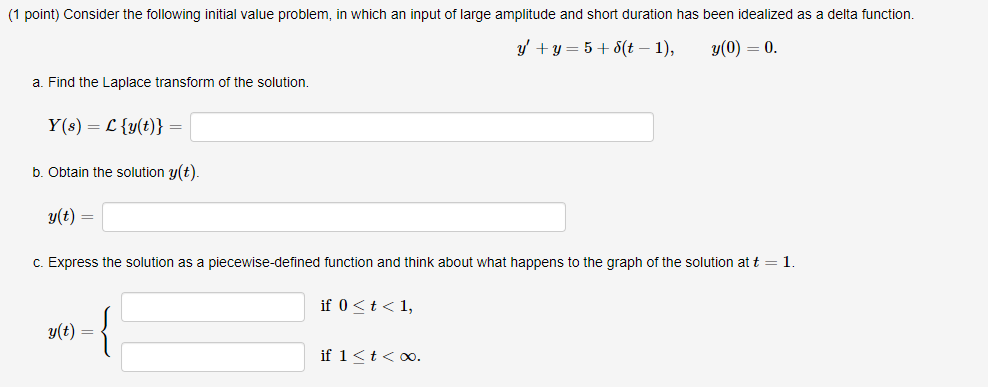### Create an Account

Already have account?

### Forgot Your Password ?

Home / Questions / (1 point) Consider the following initial value problem, in which an input of large amplitu...

# (1 point) Consider the following initial value problem, in which an input of large amplitude and short duration has been idealized as a delta function. y +y=5+(t – 1), y(0) = 0. a. Find the Laplace tr

(1 point) Consider the following initial value problem, in which an input of large amplitude and short duration has been idealized as a delta function. y +y=5+(t – 1), y(0) = 0. a. Find the Laplace transform of the solution. Y(s) = {{x(t)} = b. Obtain the solution y(t). g(t) = c. Express the solution as a piecewise-defined function and think about what happens to the graph of the solution at t = 1. if 0Apr 14 2021 View more View Less

#### Answer (Solved)Subscribe To Get Solution